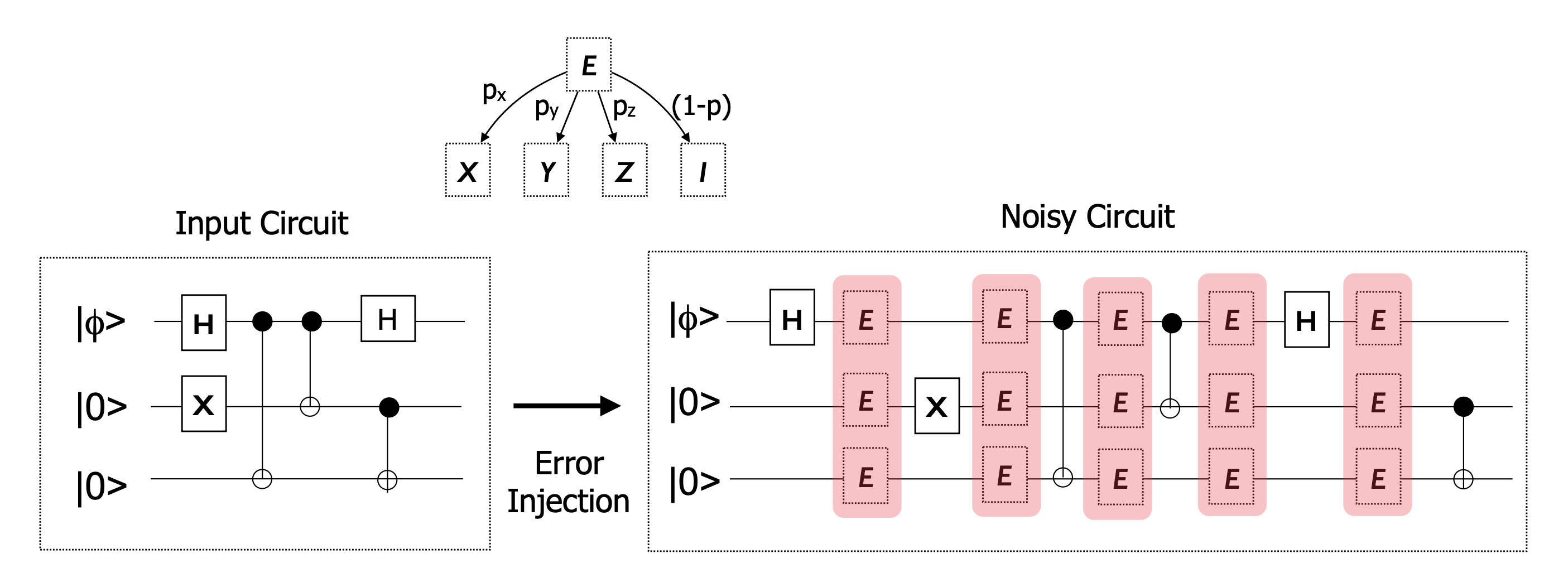# cQASM: Error models

## Introduction

By default the QX Simulator executes the circuits using perfect qubits and perfect gates, i.e. without any noise or decoherence. However real-word qubit implementations suffers from decoherence and circuits are realised using imperfect gates introducing “noisy” operations. Finally, the qubits are not perfectly isolated from the surrounding environment. This imperfect isolation is an additional noise source which contribute to the introductions of errors into the circuits. The QX Emulator currently implements one error model: the symmetric depolarising channel. A second model is under construction: the pauli-twirling approximation.

## Depolarizing channel

Selecting the depolarizing channel error model introduces a random error inbetween each operation on a qubit. These errors are injected in a form of bit-flips (x error), phase-flips (z error) or both at the same time (y error). QX Emulator implements a “Symmetric Depolarizing Channel” using equal probabilities for x, y and z errors.

The error injection process is depicted in the following figure which illustrate how errors are injected in a perfect circuit to produce a noisy circuit.Schematic of the injection of errors. Notice that an error is introduced after after operation, even in between operations that are visually parallel. Taken from 'QX Quantum Code 0.1 User Manual' by Nader Khammassi.

Below is an example of a simple Bell state experiment using the depolarizing channel error model with the per-operation error probability set to 0.001. Note that the command error_model always has to come directly after the qubits definition.

        
version 1.0

qubits 2

error_model depolarizing_channel, 0.001

prep_z q[0:1]

H q
CNOT q, q

measure_all



## Non-deterministic algorithm

Note that in the example above we added a measure instruction at the end of the algorithm. This is because the introduction of the errors in the algorithm renders the algorithm non-deterministic. For non-deterministic algorithms me must always include a measure instruction at the end in order to get meaningful results, see optimization of simulations.